#### 8.EE.8.c Analyze and solve linear equations and pairs of simultaneous linear equations: Solve real-world and mathematical problems leading to two linear equations in two variables.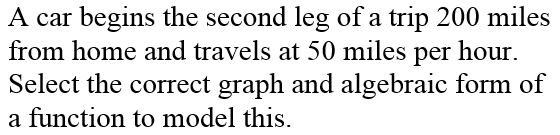Edit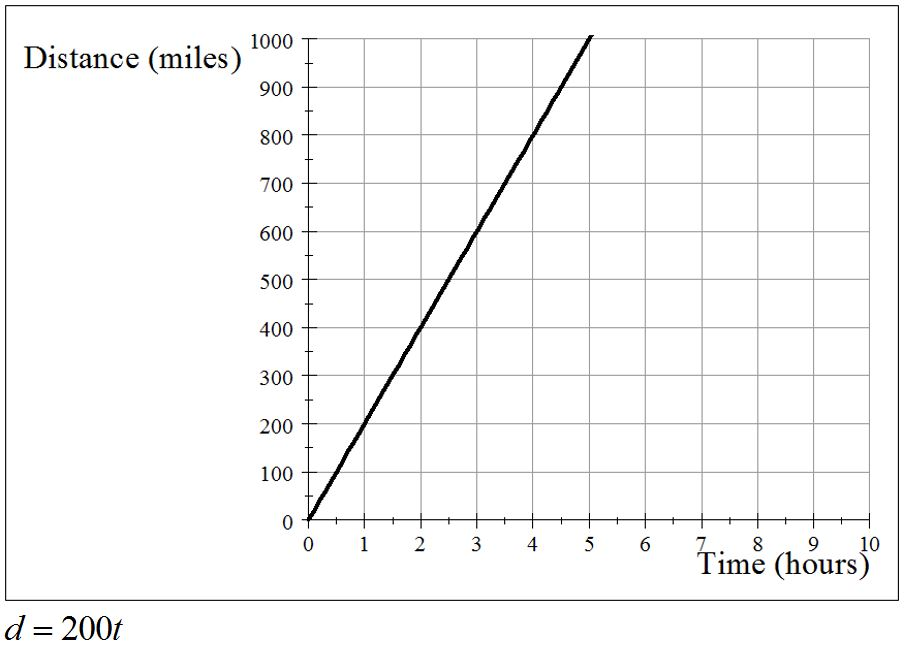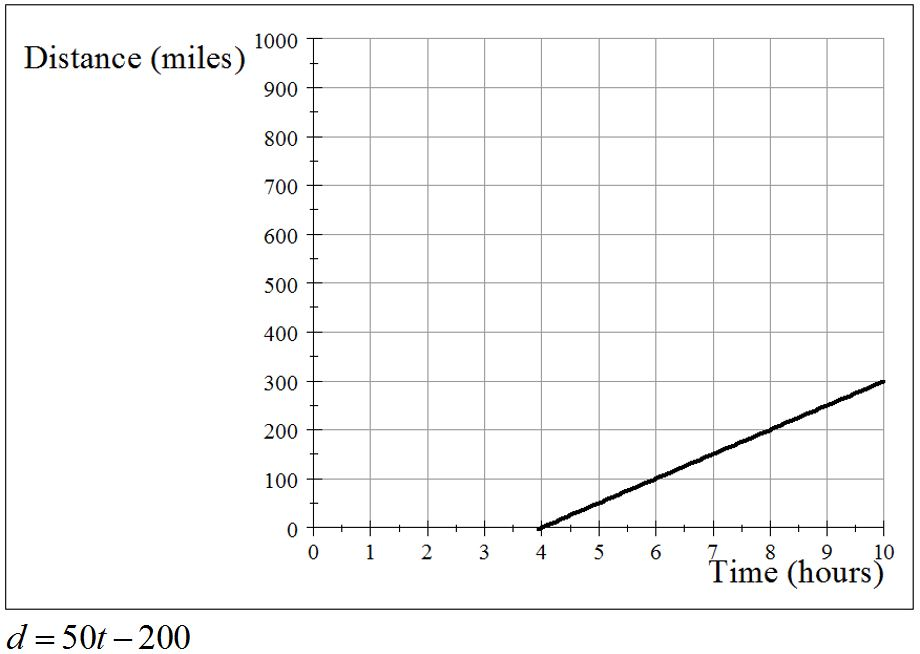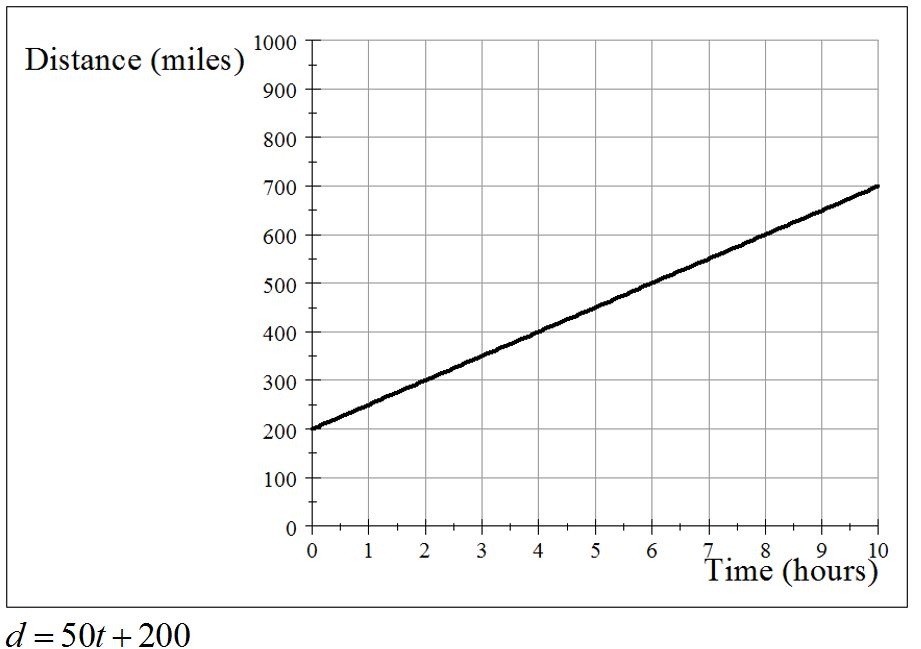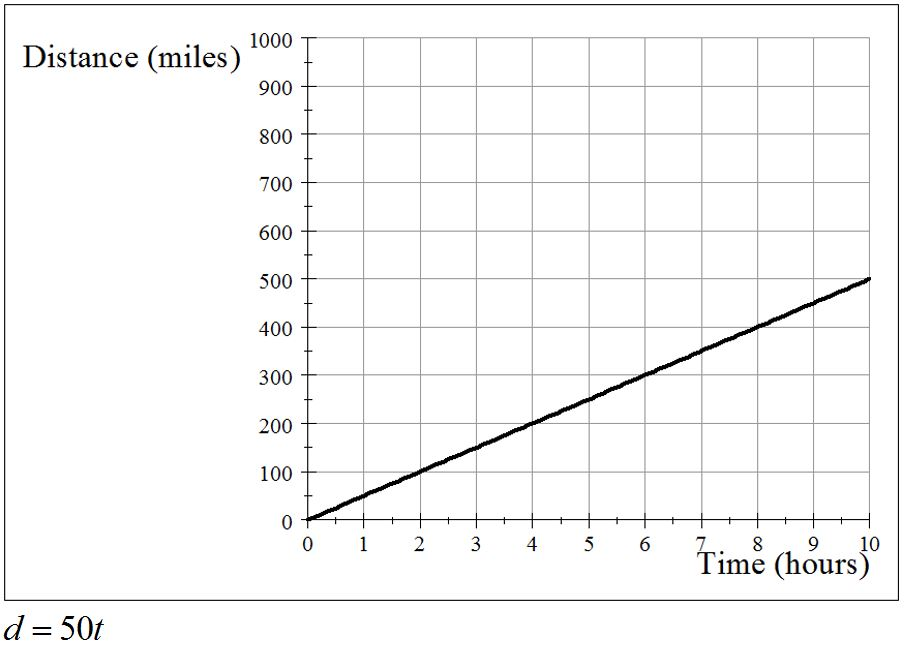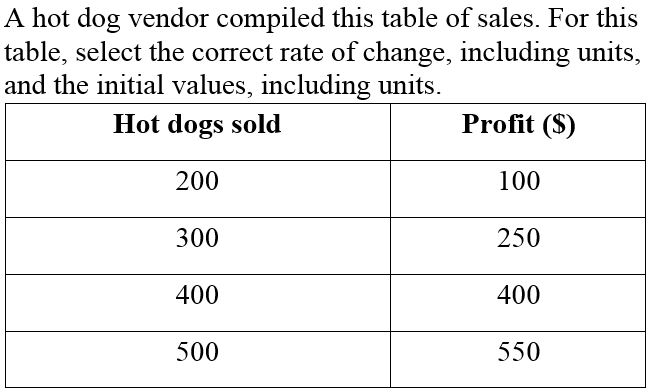Edit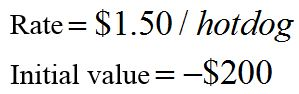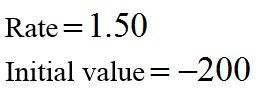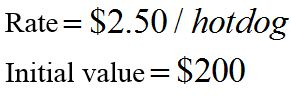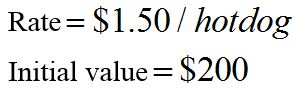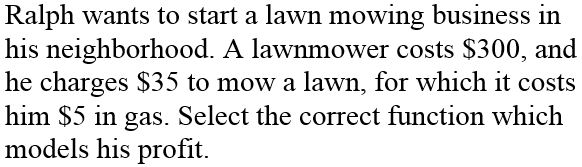Edit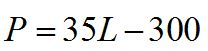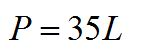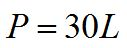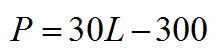Edit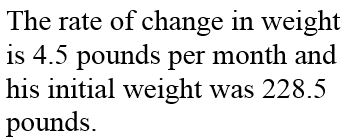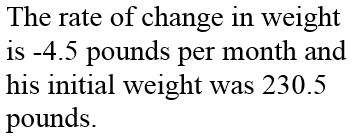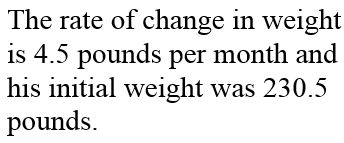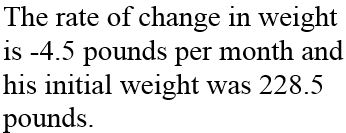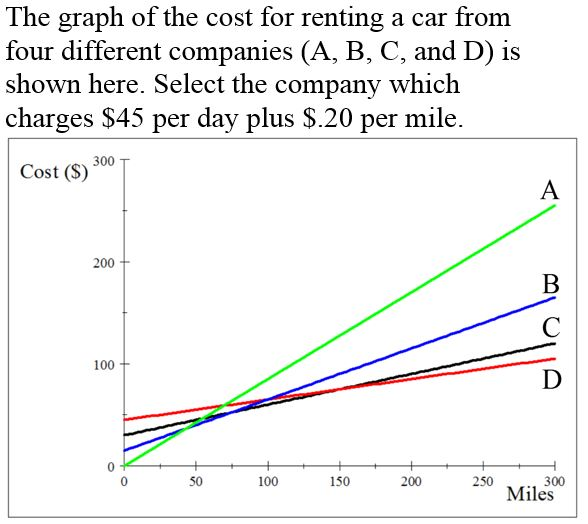Edit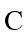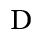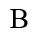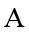Non-profit Tax ID # 203478467